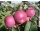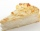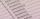# Unknown number 23

Find 2/3 of unknown number, which is two thirds of the 99.

Result

x =  44

#### Solution:Leave us a comment of example and its solution (i.e. if it is still somewhat unclear...):

Showing 0 comments:Be the first to comment!#### To solve this example are needed these knowledge from mathematics:

Need help calculate sum, simplify or multiply fractions? Try our fraction calculator. Do you have a linear equation or system of equations and looking for its solution? Or do you have quadratic equation?

## Next similar examples:

1. Unknown numberI think the number - its sixth is 3 smaller than its third.
2. Walnutsx walnuts were in the mission. Dano took 1/4 of nuts Michael took 1/8 from the rest and John took 34 nuts. It stayed here 29 nuts. Determine the original number of nuts.
3. Fifth of the numberThe fifth of the number is by 24 less than that number. What is the number?
4. Equation 20In given equation: 8/9-4/5=2/9+x, find x
5. Three monksThree medieval monks has task to copy 600 pages of the Bible. One rewrites in three days 1 page, second in 2 days 3 pages and a third in 4 days 2 sides. Calculate for how many days and what day the monks will have copied whole Bible when they begin Wednesd
6. Sales storesThe first sales store passed the 1/3 and the second 2/5 of the total amount of goods.In third store passed the 2/3 rest of the goods. The remaining 40 kg of goods put into fourth store. How many kilograms of goods pass to a third store?
7. MidnightHow many hours are, if the time that elapsed since 8:00 is 2/5 of the time that will past till midnight?
8. Mom and daughterMother is 39 years old. Her daughter is 15 years. For many years will mother be four times older than the daughter?
9. ThereThere are 32 trees of three species in the orchard. 25% cherries, three eighty pears, others plums. How many plums are in the orchard?
10. PearsThere were pears in the basket, I took two-fifths of them, and left six in the basket. How many pears did I take?
11. Supermarket 2A supermarket had a buko pie sale. In the morning 2/3 of the pies were sold and in the afternoon 1/6 of the pies were sold. If 150 pies were left, how many pies had been sold? Show your solution.
12. Simple equation 6Solve equation with one variable: X/2+X/3+X/4=X+4
13. Simple equation 5Solve equation with fractions: X × 3/8 = 1/2
14. EqnSolve equation with fractions: 2x/3-50=40+x/4
15. School marksBoris has a total of 22 marks. Ones have 3 times less than threes. There are two twos. How many has ones and threes?
16. Cakes Z8-I-5Mom brought 10 cakes of three types: kokosek was less than laskonek and most were caramel cubes. John chose two different kinds of cakes, Stephan did the same and for Margerith leave only the cakes of the same type. How many kokosek, laskonek and caramel c
17. PipeSteel pipe has a length 2.5 meters. About how many decimetres is 1/3 less than 4/8 of this steel pipe?